# ML Aggarwal Class 7 Solutions for ICSE Maths Chapter 9 Linear Equations and Inequalities Ex 9.1

## ML Aggarwal Class 7 Solutions for ICSE Maths Chapter 9 Linear Equations and Inequalities Ex 9.1

Solve the following (1 to 9) equations:
Question 1.
(i) 2 (3 – 2x) = 13
(ii) $$\frac { 3 }{ 5 }$$ y – 2 = $$\frac { 7 }{ 10 }$$
Solution: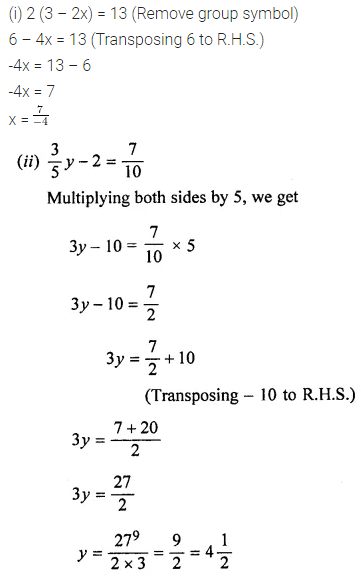Question 2.Solution: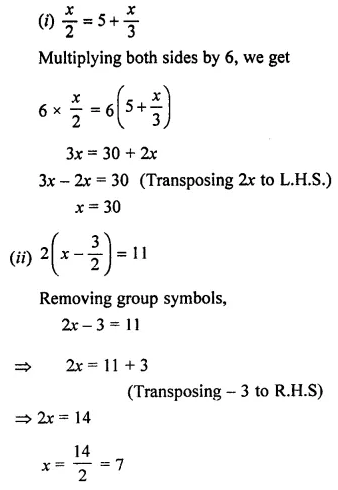Question 3.
(i) 7(x – 2) = 2 (2x – 4)
(ii) 21 – 3(x – 7) = x + 20
Solution:Question 4.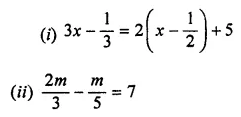Solution: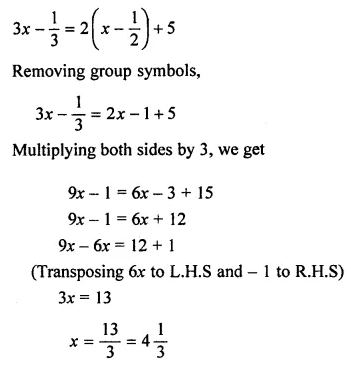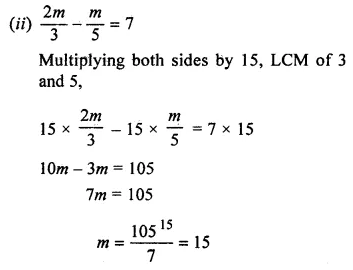Question 5.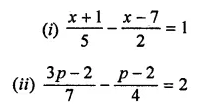Solution: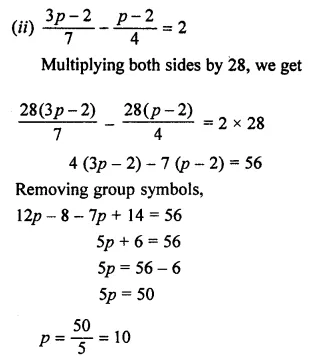Question 6.Solution:Question 7.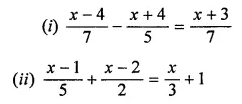Solution: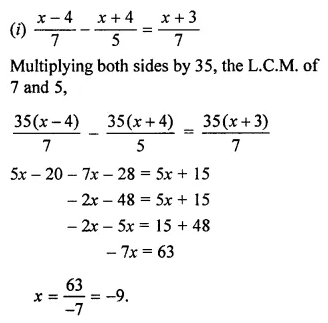Question 8.
(i) y + 1.2y = 4.4
(ii) 15% of x = 21
Solution: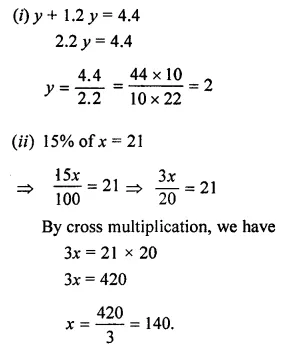Question 9.
(i) 2p + 20% of (2p – 1) = 7
(ii) 3 (2x – 1) + 25% of x = 97
Solution: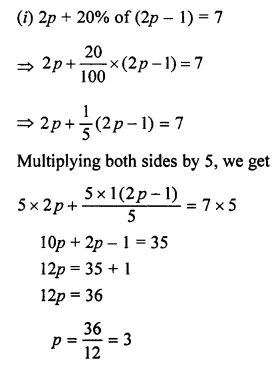Question 10.
Find the value of p if the value of x4 – 3x3 – px – 5 is equal to 23 when x = -2.
Solution: